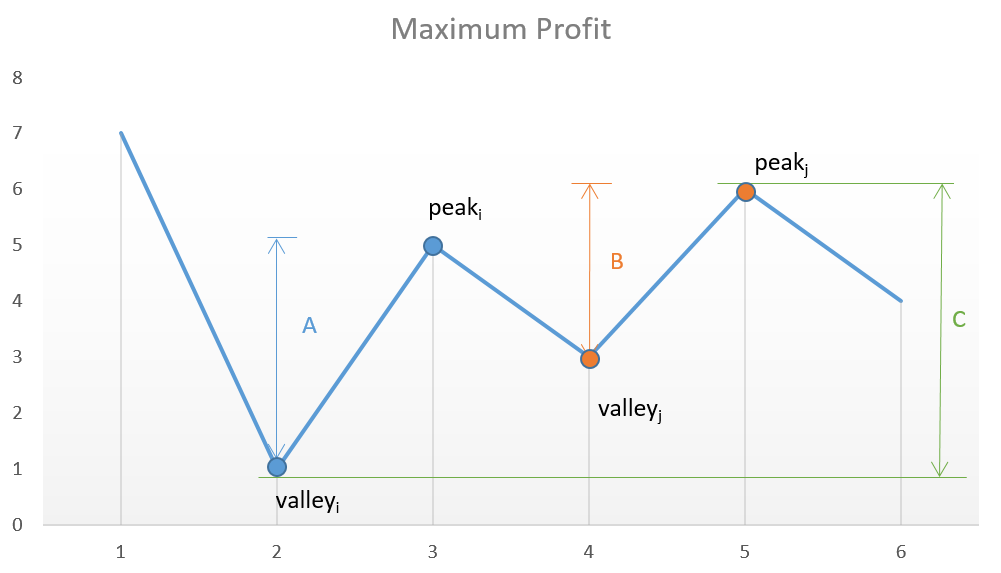# 买卖股票的最佳时机 II Best Time to Buy and Sell Stock II

## 题目

Say you have an array for which the ith element is the price of a given stock on day i.

Design an algorithm to find the maximum profit. You may complete as many transactions as you like (i.e., buy one and sell one share of the stock multiple times).

Note: You may not engage in multiple transactions at the same time (i.e., you must sell the stock before you buy again).

Example 1:

``````Input: [7,1,5,3,6,4]
Output: 7
``````

Explanation: Buy on day 2 (price = 1) and sell on day 3 (price = 5), profit = 5-1 = 4. Then buy on day 4 (price = 3) and sell on day 5 (price = 6), profit = 6-3 = 3.

Example 2:

``````Input: [1,2,3,4,5]
Output: 4
``````

Explanation: Buy on day 1 (price = 1) and sell on day 5 (price = 5), profit = 5-1 = 4. Note that you cannot buy on day 1, buy on day 2 and sell them later, as you are engaging multiple transactions at the same time. You must sell before buying again. Example 3:

``````Input: [7,6,4,3,1]
Output: 0
``````

Explanation: In this case, no transaction is done, i.e. max profit = 0.

## 思路&题解``````public int maxProfit(int[] prices) {
int sum = 0;
for(int i = 1; i<prices.length; i++) {
int diff = prices[i] - prices[i-1];
if(diff > 0){
sum +=diff;
}
}
return sum;
}
``````